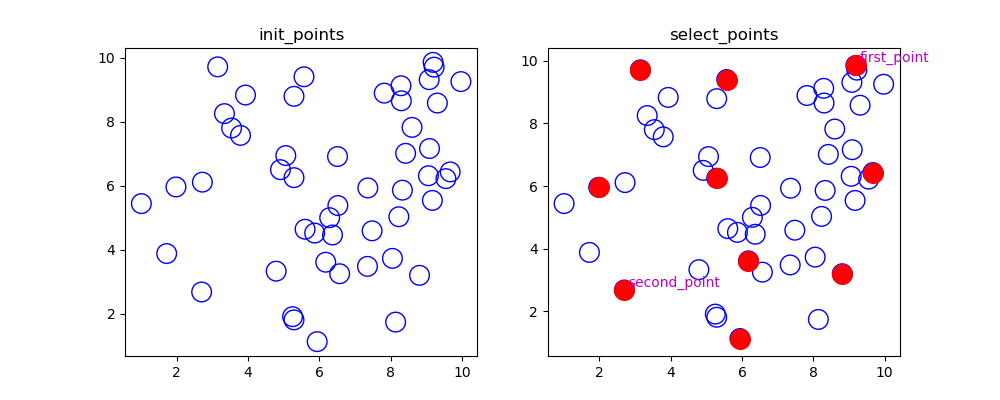## 【FPS】最远点采样Python实现_梦醒时分1218的博客-程序员宝宝

### 文章目录

\quad 在 PointNet++ 中用到了FPS(Farthest Point Sampling) 最远点采样法，该方法比随机采样的优势在于它可以尽可能的覆盖空间中的所有点。

## 1. FPS算法实现步骤

\quad 假设点集中共有 N 0 N_{0} 个点，需要采样 N 1 N_{1} 个点

step1 \textbf{step1}

\quad 在点集 S 0 S_{0} 中随机选一个点 p 0 p_{0} ,并且计算点 p 0 p_{0} S 0 S_{0} 中剩下所有点的距离，得到距离最大的点 p 1 p_{1} 。将 p 0 , p 1 p_{0},p_{1} S 0 S_{0} 中移除，加入点集 S 1 S_{1} 中，此时 S 1 = { p 0 , p 1 } S_{1} = \left\{ p_{0},p_{1}\right\}

step2 \textbf{step2}

\quad 首先计算 S 1 S_{1} 中的每个点与 S 0 S_{0} 中的每个点的距离，取最小值，如图: m i n { l 0 , l 1 } min \left\{l_{0},l_{1}\right\}\quad 计算完 S 1 S_{1} 中的每个点到 S 0 S_{0} 中的每个点的距离，并取最小值之后，再将所有值取最大值：
m a x { m i n { l i 0 , l i 1 } } , i = 1 , 2... N − 2 max\left\{ min\left\{l_{i0},l_{i1}\right\} \right\},i = 1,2...N-2

\quad 之后将该点 p 2 p_{2} ，从 S 0 S_{0} 中移除，加入 S 1 S_{1} ，此时 S 1 = { p 0 , p 1 ， p 2 } S_{1} = \left\{ p_{0},p_{1}，p_{2}\right\} S 1 S_{1} 中已经有3个点了。

step3 \textbf{step3}

\quad 循环执行 step2 \textbf{step2} ，直到 S 1 S_{1} 中的点为 N 1 N_{1} 个即可。

## 2. Python实现

import random
import math
import matplotlib.pyplot as plt

M = 50 # 所有的点数量
N = 10 # fps采样点数量
x = []
y = []
xy = []

# 生成数据
random.seed(0)
for _ in range(M):
px = round(random.uniform(1, 10), 2)  # 生成随机数，浮点类型 控制2位精度
py = round(random.uniform(1, 10), 2)
x.append(px)
y.append(py)
xy.append((px, py))

# 显示生成的点
plt.figure(figsize=(10, 4))
plt.subplot(121)
plt.title('init_points')
plt.scatter(x, y, marker='o', c='', edgecolors='b', s=200)

select = []
rest = [num for num in range(0, M)]

max_dist = -99999
farthest_point = 99999

# step 1 随机选择一个点
random.seed(1)
ind = random.randint(0, M - 1)
select.append(ind)
rest.remove(ind)
# print('select', select)
# print('rest', rest)

# 计算距离,找到距离第一个点最远的点
for i in range(len(xy)):
if i != ind:
length = math.sqrt(math.pow(abs(xy[ind] - xy[i]), 2) + math.pow(abs(xy[ind] - xy[i]), 2))
if length > max_dist:
max_dist = length
farthest_point = i
select.append(farthest_point)
rest.remove(farthest_point)
print('select', select)
print('rest', rest)

# print(dist)
# print(max_dist)
# print(farthest_point)

# step 2 先min,再max
print('----after----')
while len(select) < N:
min_length = []
max_dist = -99999

for i in range(len(rest)):
min_dist = 99999

for j in range(len(select)):
length = math.sqrt(
math.pow(abs(xy[rest[i]] - xy[select[j]]), 2) + math.pow(abs(xy[rest[i]] - xy[select[j]]),
2))
if length < min_dist:  # 先取最小
min_dist = length

min_length.append((rest[i], min_dist))

if list(min_length[i]) > max_dist:  # 再取最大
max_dist = list(min_length[i])
farthest_point = list(min_length[i])

# print(min_length)
# print(max_dist)
# print(farthest_point)
# print('------------------------')
select.append(farthest_point)
rest.remove(farthest_point)

print('select', select)
print('rest', rest)

# fps点
new_x = []
new_y = []
for i in range(len(select)):
new_x.append(xy[select[i]])
new_y.append(xy[select[i]])

plt.subplot(122)
plt.title('select_points')
plt.scatter(x, y, marker='o', c='', edgecolors='b', s=200)  # edgecolors是控制圆圈的边缘颜色，c是控制圆心的颜色，c=''就是空心
plt.scatter(new_x, new_y, marker='o', c='red', edgecolors='r', s=200)
plt.text(new_x + 0.1, new_y + 0.1, "first_point", size=10, color="m")
plt.text(new_x + 0.1, new_y + 0.1, "second_point", size=10, color="m")
# plt.savefig("FPS.png")
plt.show()


## 3. 结果显示### CTex+WinEdt10.2安装详细教程与破解_weixin_30885111的博客-程序员宝宝

Latex作为目前最好用的文档编排工具，以前只是简单会一点，现在也已经忘得差不多了。因为写小论文的需要，打算重新开始学习，以前用的是Texlive和Texmaker，这次经过师兄的推荐，还是打算选择CTex套装，但自己把WinEdt7.0换成了WinEdt10.2，并完美注册码破解。经过两个多小时的安装，总算是搞定了，中间卸载过两次，比较丢人，虽然现在看来很简单，但也花了不少功夫，因此总结一下，希...

### VARCHART XGantt系列教程：如何在分组视图中更好地显示节点_vc++中varchart xgantt控件使用教程_ymy_666666的博客-程序员宝宝

VARCHART XGantt是一款功能强大的甘特图控件，其模块化的设计让您可以创建满足需要的应用程序。XGantt可用于.NET，ActiveX和ASP.NET应用程序，可以快速、简单地集成到您的应用程序中，帮助您识别性能瓶颈、避免延迟以及高效利用资源，使复杂数据变得更加容易理解。本文主要介绍如何在分组视图中更好地显示节点，有任何建议或提示请在下方评论区留言。在甘特图中活动通常以组的...

### SSD: Single Shot MultiBox Detection 自己的一些理解_写代码_不错哦的博客-程序员宝宝

SSD: Single Shot MultiBox Detection论文翻译Pytorch 代码根据这个人的文章，可以把 SSD 代码跑起来：https://zhuanlan.zhihu.com/p/92154612https://blog.csdn.net/qq_30815237/article/details/90292639SSD算法是一种直接预测目标类别和bounding box的多目标检测算法。针对不同大小的目标检测，传统的做法是先将图像转换成不同大小（图像金字塔

### VMware vCenter Converter 的p2v实际应用（备份系统功能）_晚风_END的博客-程序员宝宝

VMware vCenter Converter 的p2v实际应用（备份系统功能）一、VMware vCenter Converter版本及组成1、版本vCenter Converter分Enterprise和Standalone 2个版本，前者属于vSphere Enterprise授权，后者是免费版；2者功能上几乎相同，前者安装后所有操作界面集成在vCenter中，后者则是单独的操作界面；而Standalone支持迁移最新的Window...

### Android EditText使用_a13069730106的博客-程序员宝宝

Android EditText 进阶使用.

### linux系统hostapd强制使用40MHz频宽_hostapd he_phy_channel_width_set_李迟的博客-程序员宝宝

linux系统实现WIFI热点服务一般是使用hostapd来完成的。802.11n标准可以使用20MHz、40MHz频宽，这在hostapd配置文件中可以指定。另外，还可以使用Short GI使速率提升10%。